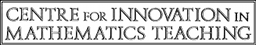GCSE Sample Test
Paper 1
How would you like to complete the test?
Check question by question
Check the test at the end
marks

How many centimetres are there in 2.9 metres?

0.029
0.29
29
290
[1 mark] ?
2.9 metres = 2.9 × 100 cm = 290 cm
marks

Which of these is the net of a cube?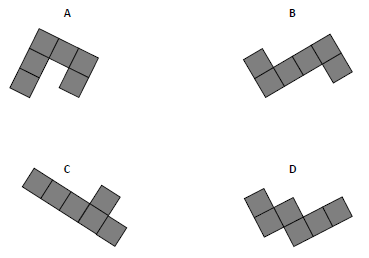[1 mark] ?
Only B will form a complete cube
marks

Which of these fractions is not equivalent to

[1 mark] ?
= but all others are equivalent to ; that is × = , × = and × =
marks

Simplify 4𝑎 − (𝑎 − 7)

3𝑎 − 7
5𝑎 − 7
5𝑎 + 7
3𝑎 + 7
[1 mark] ?
4𝑎 − (𝑎 − 7) = 4𝑎 − 𝑎 + 7 = 3𝑎 + 7
marks

Complete the table.

 Minutes Hours 15 40 1
[2 marks] ?
= ; 1 = and hour = × 60 min = 80 mins
marks

Here are some numbers.

10.4 12.6 15.6 8.6 13.4 17.4

Write the numbers in pairs so that the sum of the numbers in each pair is the same.

and

and

and

[2 marks] ?
Put the numbers in order: 17.4, 15.6, 13.4, 12.6, 10.4, 8.6 and then take the largest and smallest (first and last) and continue in this way
marks

This triangle is drawn accurately.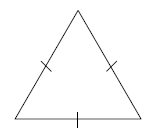What type of triangle is it?

Tick all correct boxes.

acute-angled
obtuse-angled
equilateral
isosceles
scalene
[3 marks] ?
acute-angled (all angles are 60°), equilateral (all angles equal and all sides equal), isosceles (two sides equal) but NOT obtuse (no angle larger than 90°) and NOT scalene (as this has to have 3 unequal sides)
marks
Work out 49% of 500
[2 marks] ?
49% of 500 means × 500 = 49 × 5 = 245
marks
Write 120 g as a fraction of 3 kg Give your answer in its simplest form.
[2 marks] ?
3kg = 3000g so fraction is = = =
marks
Here are some properties of numbers. A Even B Odd C Prime D Square E Two-digit
(a)

Which two properties does the number 9 have?
Circle the correct letters.

A
B
C
D
E
[1 mark] ?
9 is ODD and SQUARE (as 9 = 3 × 3 = 32)
(b)

Can one number have all of the properties?
Tick a box.

Yes
No
Cannot tell
[1 mark] ?
No, as any number is either ODD or EVEN but not both
(c)

Write down a number that has properties B, D and E.

[2 marks] ?
25, 49 and 81 are all ODD (B) and SQUARE (C) and TWO-DIGIT (E)
marks

Chris has five coins in his pocket.

If he picks four of the coins

the most he could pick is £5.20
the least he could pick is £3.30

How much money does he have altogether?

£ [4 marks] ?
The difference between the sum of the highest 4 coins and sum of the lowest 4 coins is the difference between the highest and lowest coins; this is £5.20 – £3.30 = £1.90 so the highest coin has to be £2 and the lowest 10p; hence total is £5.20 + 10p = £5.30
marks

Here are three expressions.

ab ab

When 𝑎 = −2 and 𝑏 = 8 which expression has the smallest value and what is its value?

(i)
Expression:
(ii)
Value: ?
= = − ; 𝑎 − 𝑏 = −2 − 8 = −10 ; 𝑎𝑏 = (−2) × 8 = −16. The smallest value is –16.
[2 marks]
marks

The table shows the ratio of teachers to children needed for two activities.

 teachers : children Climbing 1 : 3 Walking 1 : 11
(a)

There are 7 teachers to take children climbing.
What is the greatest number of children that can go climbing?

[1 mark] ?
7 × 3 = 21 so greatest number of children that can be taken climbing is 21
(b)

49 children want to go walking.
What is the smallest number of teachers needed?

[1 mark] ?
49 ÷ 11 = 4 (and remainder 5) so at least 5 teachers are needed
marks

Shape S is a rectangle.
A smaller shape is cut from S to form shape C.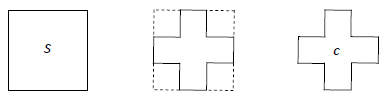Which one of these statements is true?
Tick a box.

The perimeter of S is longer than the perimeter of C
The perimeter of S is the same as the perimeter of C
The perimeter of S is shorter than the perimeter of C
It is not possible to tell which perimeter is longer
[1 mark] ?
The dotted parts in the second shape have the same length as the inside parts of the new shape so the total perimeter has not changed (even though the area is clearly reduced)
marks

Textbooks are stored on two shelves.
Each shelf is 0.84 metres long.
Each textbook is 30 millimetres wide.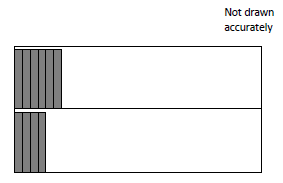(a)

What is the total width in metres of the 60 textbooks?

m [2 marks] ?
60 mm × 30 = 1800 mm = 1.80 m
(b)

Can 60 textbooks be stored on these two shelves?

[1 mark] ?
2 × 0.84 m = 1.68 m < 1.80 m (Note that the symbol < means “less than” )
marks

All tickets for a concert are the same price.
Amy and Dan pay £63 altogether for some tickets.
Amy pays £40.50 for 9 tickets.
How many tickets does Dan buy?

[4 marks]
£40.50 ÷ 9 = £4.50 so each ticket costs £4.50. So 2 tickets will cost £9 and 14 tickets will cost 7 x £9 = £63
marks

Here is the graph of 𝑦 = 4 − 𝑥 for values of x from 0 to 4.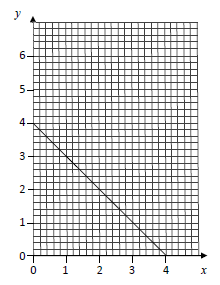On the same grid, consider the position of the graph of 𝑦 = 𝑥 − 2 for values
of x from 2 to 5 and hence solve the simultaneous equations
𝑦 = 4 − 𝑥 and 𝑦 = 𝑥 − 2

(i)
x =
(ii)
y = ?
The lines intersect (see diagram) at x = 3 and y = 1 (you can check these answers by substituting into the two equations: when 𝑥 = 3, 𝑦 = 4 − 𝑥 = 4 − 3 = 1 and 𝑦 = 𝑥 − 2 = 3 − 2 = 1)
[2 marks]
marks

The table shows the sales of food and drink for three days at a café.

 Day Sales of food (£) Sales of drink (£) Friday 35 15 Saturday 24 44 Sunday 30 33

Hannah uses this information to draw a composite bar chart.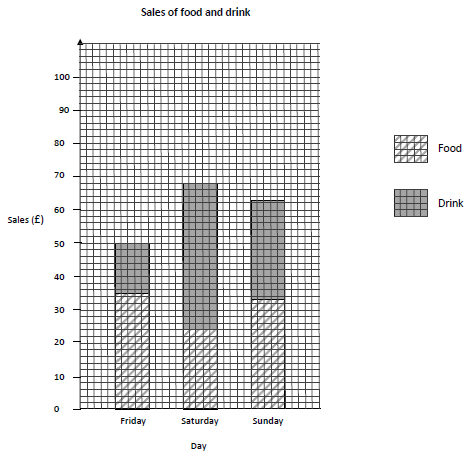(i)

Friday:

Is there a mistake in the FOOD bar?

Is there a mistake in the DRINK bar?

(ii)

Saturday:

Is there a mistake in the FOOD bar?

Is there a mistake in the DRINK bar?

?
For DRINK, the diagram shows 64 – 24 = 40 sales but it should have been 44
(iii)

Sunday:

Is there a mistake in the FOOD bar?

Is there a mistake in the DRINK bar?

?
The FOOD and DRINK are reversed
[3 marks]
marks

Lisa wants to buy a laptop for £345.
Each week
her pay is £120
she saves 25% of this pay.
For how many more weeks must she save?

[3 marks] ?
£345 – £115 = £230 and × £120 = £30. 7 × 30 = 210 but 8 × 30 = 240 so she must save for 8 weeks
marks
(a)

x and y are whole numbers.

𝑥 > 50

𝑦 < 20

Work out the smallest possible value of 𝑥 − 𝑦

[2 marks] ?
Smallest x – largest y = 51 – 19 = 32
(b)

x and y are whole numbers.

𝑥 ≥ 60

𝑦 > 50

Work out the smallest possible value of 𝑦 + 𝑥

[2 marks] ?
Smallest x + smallest y = 60 + 51 = 111
marks
(a)

Work out 3.4 × 0.003

[1 mark] ?
3.4 × 3 = 10.2 so 3.4 × 0.003 = 0.0102
(b)

Work out 4.7 × 10−3

[1 mark] ?
4.7 × 10−3 = = 0.0047
(c)

Write 347 200 in standard form.

× 10 [1 mark] ?
347 200 = 3.472 × 10 000 = 3.472 × 105
marks

The diagram shows information about the scores of Year 5 students in a spelling test.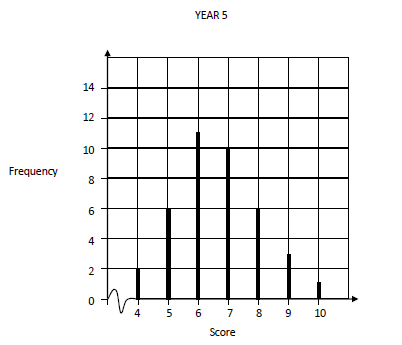(a)

A student is chosen at random from Year 5.
Work out the probability that the student’s score was the mode for Year 5.

[3 marks] ?
Mode was score 6; the total number of students = 2 + 6 + 11 + 10 + 6 + 3 + 1 = 39 giving p = =

The diagram shows information about the scores of Year 6 students in the same test.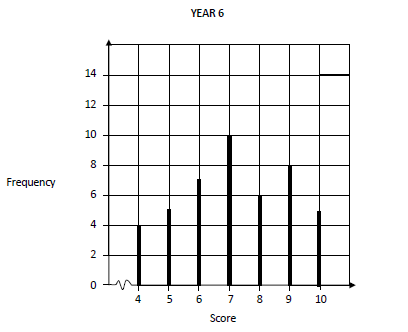(b)

Do the Year 6 students have more consistent scores than the Year 5 students.

YES
NO
CANNOT TELL
[1 mark]
There is greater variation in Year 5 than Year 6; the frequency range is 1 to 11 for Year 5 but only 4 to 10 for Year 6
(c)

Laura is one of the 45 students in Year 6.
Her score was the same as the median score for her year.
Work out her score.

[3 marks] ?
The median score for Year 6 will be the 23rd when in order; there are 16 with scores 4, 5 and 6 so the median is in the group scoring 7.
marks

Kelly is trying to work out the two values of x for which 2x2x3 = 0
Her values are 2 and −2

(a)

Are her two values correct?

[1 mark]
(b)

If incorrect, find the correct value.

x = or [2 marks] ?
2𝑥2 − 𝑥3 = 2 × 𝑥2 − 𝑥 × 𝑥2 = (2 − 𝑥)𝑥2 = 0 when (2 – x) = 0 or 𝑥2 = 0; so 𝑥 = 2 or 𝑥 = 0
marks

The diagram shows a semicircle of radius 8 cm.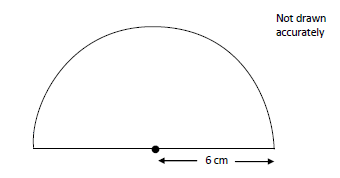Work out the area of the semicircle.

𝜋 [2 marks] ?
Area of circle = 𝜋𝑟2 so area of semicircle = 𝜋𝑟2 = 𝜋 6 × 6 = 18𝜋
marks

Work out 3 × 2

[2 marks] ?
3 × 2 = × = × = = 8
marks

Solve 3𝑥 − 4 ≤ 16 − 𝑥

x [2 marks] ?
Add 𝑥 to both sides to give 4𝑥 – 4 ≤ 16 and now add 4 to both sides, 4𝑥 ≤ 20 and divide both sides to obtain the solution 𝑥 ≤ 5.
marks

The nth term of a sequence is 3n + 2

The nth term of a different sequence is 4n − 3

Work out the two numbers that are

in both sequences

and

between 10 and 30

and [4 marks] ?
The first sequence has numbers 5, 8, 11, 14, 17, 20, 23, 26, 29,... so between 10 and 30, we have 11, 14, 17, 20, 23, 26, 29. The second sequence is 1, 5, 9, 13, 17, 21, 25, 29 so between 10 and 30, we have 13, 17, 21, 25, 29. Numbers common to these two sequences and 17 and 29.
marks

White paint costs £2.60 per litre.

Blue paint costs £3.20 per litre.

White and blue paint are mixed in the ratio 3 : 2.

Work out the cost of 20 litres of the mixture.

£ [4 marks] ?
3 litres of WHITE paint and 2 litres of BLUE paint give 5 litres of the mixture, costing 3 × £2.60 + 2 × £3.20 = £7.80 + £6.40 = £14.20; so for 20 litres, it costs 4 × £14.20 = £58.80
marks

Here is the sketch of a triangle.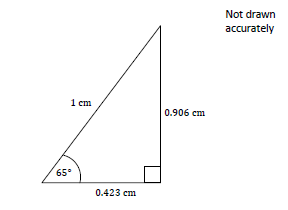Distances are given to 3 decimal places.

(a)

Circle the value of cos 65° to 3 decimal places.

0.906
0.423
0.014
0.577
[1 mark] ?
cos65° = = 0.423
(b)

Work out the value of x.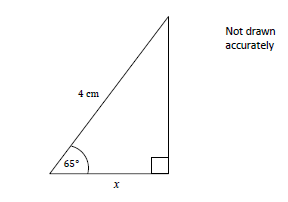[2 marks] ?
𝑥 = 4 × 0.423 = 1.692 ≈ 1.69
marks

ABCH is a square.
HCFG is a rectangle.
CDEF is a square.

They are joined to make an L-shape.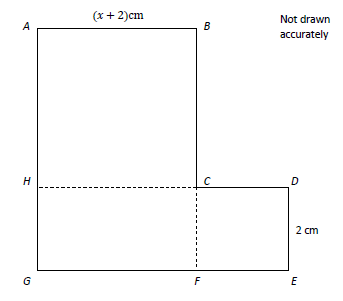The total area of the L-shape, in cm2, is of the form ax2 + bx + c where a, b and c are numbers.

What are the values of a, b and c ?

(i)
a =
(ii)
b =
(iii)
c = ?
Area of ABCH + HCFG + CDEF = (𝑥 + 2)2 + 2(𝑥 + 2) + 4 = 𝑥2 + 4𝑥 + 4 + 2𝑥 + 4 + 4 = 𝑥2 + 6𝑥 + 12; hence 𝑎 = 1, 𝑏 = 6, 𝑐 = 12
[3 marks]
Test check
Total: - of marks